# DBSCAN Clustering in ML | Density based clustering

• Difficulty Level : Medium
• Last Updated : 15 Jun, 2022

Clustering analysis or simply Clustering is basically an Unsupervised learning method that divides the data points into a number of specific batches or groups, such that the data points in the same groups have similar properties and data points in different groups have different properties in some sense. It comprises many different methods based on differential evolution.
E.g. K-Means (distance between points), Affinity propagation (graph distance), Mean-shift (distance between points), DBSCAN (distance between nearest points), Gaussian mixtures (Mahalanobis distance to centers), Spectral clustering (graph distance) etc.

Fundamentally, all clustering methods use the same approach i.e. first we calculate similarities and then we use it to cluster the data points into groups or batches. Here we will focus on Density-based spatial clustering of applications with noise (DBSCAN) clustering method.Clusters are dense regions in the data space, separated by regions of the lower density of points. The DBSCAN algorithm is based on this intuitive notion of “clusters” and “noise”. The key idea is that for each point of a cluster, the neighborhood of a given radius has to contain at least a minimum number of points.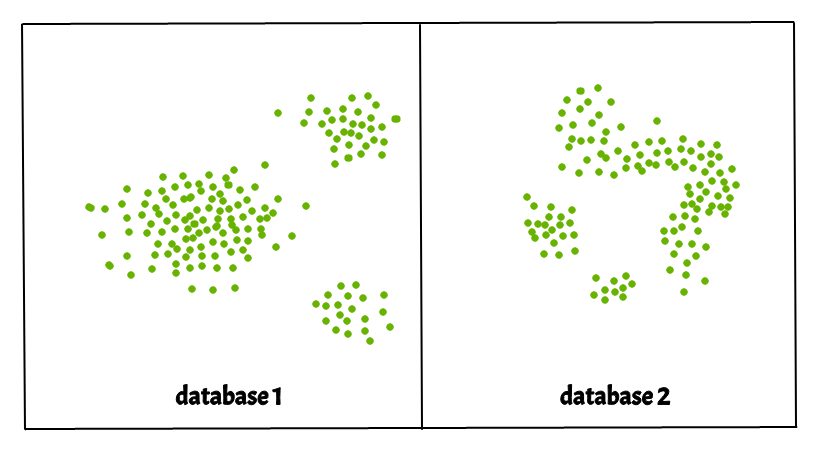Why DBSCAN?
Partitioning methods (K-means, PAM clustering) and hierarchical clustering work for finding spherical-shaped clusters or convex clusters. In other words, they are suitable only for compact and well-separated clusters. Moreover, they are also severely affected by the presence of noise and outliers in the data.

Real life data may contain irregularities, like:

1. Clusters can be of arbitrary shape such as those shown in the figure below.
2. Data may contain noise.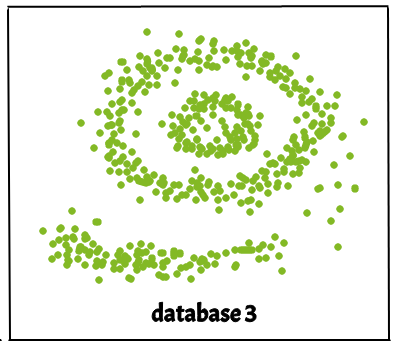The figure below shows a data set containing nonconvex clusters and outliers/noises. Given such data, k-means algorithm has difficulties in identifying these clusters with arbitrary shapes.
DBSCAN algorithm requires two parameters:

1. eps : It defines the neighborhood around a data point i.e. if the distance between two points is lower or equal to ‘eps’ then they are considered neighbors. If the eps value is chosen too small then large part of the data will be considered as outliers. If it is chosen very large then the clusters will merge and the majority of the data points will be in the same clusters. One way to find the eps value is based on the k-distance graph.
2. MinPts: Minimum number of neighbors (data points) within eps radius. Larger the dataset, the larger value of MinPts must be chosen. As a general rule, the minimum MinPts can be derived from the number of dimensions D in the dataset as, MinPts >= D+1. The minimum value of MinPts must be chosen at least 3.

In this algorithm, we have 3 types of data points.
Core Point: A point is a core point if it has more than MinPts points within eps.
Border Point: A point which has fewer than MinPts within eps but it is in the neighborhood of a core point.
Noise or outlier: A point which is not a core point or border point.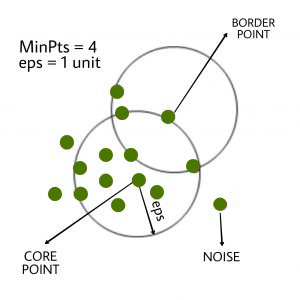DBSCAN algorithm can be abstracted in the following steps:

1. Find all the neighbor points within eps and identify the core points or visited with more than MinPts neighbors.
2. For each core point if it is not already assigned to a cluster, create a new cluster.
3. Find recursively all its density connected points and assign them to the same cluster as the core point.
A point a and b are said to be density connected if there exist a point c which has a sufficient number of points in its neighbors and both the points a and b are within the eps distance. This is a chaining process. So, if b is neighbor of c, c is neighbor of d, d is neighbor of e, which in turn is neighbor of a implies that b is neighbor of a.
4. Iterate through the remaining unvisited points in the dataset. Those points that do not belong to any cluster are noise.

Below is the DBSCAN clustering algorithm in pseudocode:

```DBSCAN(dataset, eps, MinPts){
# cluster index
C = 1
for each unvisited point p in dataset {
mark p as visited
# find neighbors
Neighbors N = find the neighboring points of p

if |N|>=MinPts:
N = N U N'
if p' is not a member of any cluster:
}```

Implementation of the above algorithm in Python :
Here, we’ll use the Python library sklearn to compute DBSCAN. We’ll also use the matplotlib.pyplot library for visualizing clusters. The dataset used can be found here.

### Evaluation Metrics

Moreover, we will use the Silhouette score and Adjusted rand score for evaluating clustering algorithms.

Silhouette score is in the range of -1 to 1. A score near 1 denotes the best meaning that the data point i is very compact within the cluster to which it belongs and far away from the other clusters. The worst value is -1. Values near 0 denote overlapping clusters.

Absolute Rand Score is in the range of 0 to 1. More than 0.9 denotes excellent cluster recovery, above 0.8 is a good recovery. Less than 0.5 is considered to be poor recovery.

Example

## Python3

 `import` `matplotlib.pyplot as plt``import` `numpy as np``from` `sklearn.cluster ``import` `DBSCAN``from` `sklearn ``import` `metrics``from` `sklearn.datasets.samples_generator ``import` `make_blobs``from` `sklearn.preprocessing ``import` `StandardScaler``from` `sklearn ``import` `datasets` `# Load data in X``X, y_true ``=` `make_blobs(n_samples``=``300``, centers``=``4``,``                       ``cluster_std``=``0.50``, random_state``=``0``)``db ``=` `DBSCAN(eps``=``0.3``, min_samples``=``10``).fit(X)``core_samples_mask ``=` `np.zeros_like(db.labels_, dtype``=``bool``)``core_samples_mask[db.core_sample_indices_] ``=` `True``labels ``=` `db.labels_` `# Number of clusters in labels, ignoring noise if present.``n_clusters_ ``=` `len``(``set``(labels)) ``-` `(``1` `if` `-``1` `in` `labels ``else` `0``)` `print``(labels)` `# Plot result` `# Black removed and is used for noise instead.``unique_labels ``=` `set``(labels)``colors ``=` `[``'y'``, ``'b'``, ``'g'``, ``'r'``]``print``(colors)``for` `k, col ``in` `zip``(unique_labels, colors):``    ``if` `k ``=``=` `-``1``:``        ``# Black used for noise.``        ``col ``=` `'k'` `    ``class_member_mask ``=` `(labels ``=``=` `k)` `    ``xy ``=` `X[class_member_mask & core_samples_mask]``    ``plt.plot(xy[:, ``0``], xy[:, ``1``], ``'o'``, markerfacecolor``=``col,``             ``markeredgecolor``=``'k'``,``             ``markersize``=``6``)` `    ``xy ``=` `X[class_member_mask & ~core_samples_mask]``    ``plt.plot(xy[:, ``0``], xy[:, ``1``], ``'o'``, markerfacecolor``=``col,``             ``markeredgecolor``=``'k'``,``             ``markersize``=``6``)` `plt.title(``'number of clusters: %d'` `%` `n_clusters_)``plt.show()` `#evaluation metrics``sc ``=` `metrics.silhouette_score(X, labels)``print``(``"Silhouette Coefficient:%0.2f"``%``sc)``ari ``=` `adjusted_rand_score(y_true, labels)``print``(``"Adjusted Rand Index: %0.2f"``%``ari)`

Output: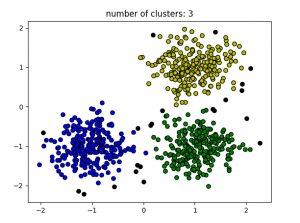```Silhouette Coefficient:0.13

Black points represent outliers. By changing the eps and the MinPts , we can change the cluster configuration.
Now the question should be raised is – Why should we use DBSCAN where K-Means is the widely used method in clustering analysis?

K-Means forms spherical clusters only. This algorithm fails when data is not spherical ( i.e. same variance in all directions).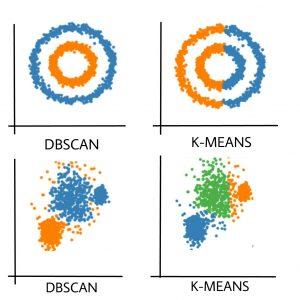K-Means algorithm is sensitive towards outlier. Outliers can skew the clusters in K-Means in very large extent.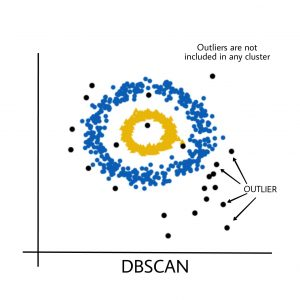K-Means algorithm requires one to specify the number of clusters a priory etc.

Basically, DBSCAN algorithm overcomes all the above-mentioned drawbacks of K-Means algorithm. DBSCAN algorithm identifies the dense region by grouping together data points that are closed to each other based on distance measurement.
Python implementation of the above algorithm without using the sklearn library can be found here dbscan_in_python

My Personal Notes arrow_drop_up